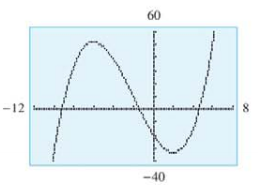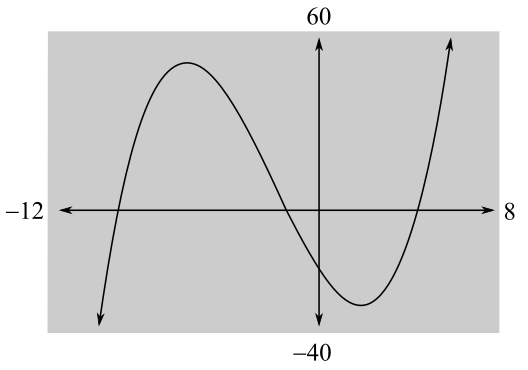Chapter 10.2, Problem 24E### Mathematical Applications for the ...

11th Edition
Ronald J. Harshbarger + 1 other
ISBN: 9781305108042

#### Solutions

Chapter
Section### Mathematical Applications for the ...

11th Edition
Ronald J. Harshbarger + 1 other
ISBN: 9781305108042
Textbook Problem

# In Problems 25 and 26, a function and its graph are given.T (a) From the graph, estimate where f " ( x )   > 0 , where f " ( x ) <   0 , and where f " ( x ) =   0 .(b) Use part (a) to decide where f ' ( x ) has its relative maxima and relative minima.(c) Verify your results in parts (a) and (b) by finding f ' ( x ) and f " ( x ) and then graphing each with a graphing utility. f ( x ) = 1 3 x 3 + 2 x 2 − 12 x − 20(a)

To determine

The region where f(x)>0, f"(x)<0 and f(x)=0 in the graph of the function f(x)=13x3+2x212x20.Explanation

Given Information:

The provided graph of the function f(x)=13x3+2x212x20 is

Explanation:

Consider the provided graph of the function f(x)=13x3+2x212x20,

Recall that if f(x)>0 and f(x) is concave up and if f(x)<0 and f(x) is concave down.

From the graph, if x>2 then graph is concave up and if x<2 then the graph is concave down and at point x=2 the graph changes its concavity

(b)

To determine

Where f(x) has relative maxima and relative minima for the provided graph of a function f(x)=13x3+2x212x20.(c)

To determine

The values of f(x) and f(x), the region where f(x)>0, f(x)<0 and f(x)=0 and where f(x) has relative maxima and relative minima for the function f(x)=13x3+2x212x20 and graph each function f(x) and f(x).

### Still sussing out bartleby?

Check out a sample textbook solution.

See a sample solution

#### The Solution to Your Study Problems

Bartleby provides explanations to thousands of textbook problems written by our experts, many with advanced degrees!

Get Started